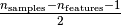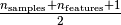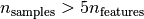This documentation is for scikit-learn version 0.16.1Other versions

If you use the software, please consider citing scikit-learn.

# Robust vs Empirical covariance estimate¶

The usual covariance maximum likelihood estimate is very sensitive to the presence of outliers in the data set. In such a case, it would be better to use a robust estimator of covariance to guarantee that the estimation is resistant to “erroneous” observations in the data set.

## Minimum Covariance Determinant Estimator¶

The Minimum Covariance Determinant estimator is a robust, high-breakdown point (i.e. it can be used to estimate the covariance matrix of highly contaminated datasets, up tooutliers) estimator of covariance. The idea is to findobservations whose empirical covariance has the smallest determinant, yielding a “pure” subset of observations from which to compute standards estimates of location and covariance. After a correction step aiming at compensating the fact that the estimates were learned from only a portion of the initial data, we end up with robust estimates of the data set location and covariance.

The Minimum Covariance Determinant estimator (MCD) has been introduced by P.J.Rousseuw in .

## Evaluation¶

In this example, we compare the estimation errors that are made when using various types of location and covariance estimates on contaminated Gaussian distributed data sets:

• The mean and the empirical covariance of the full dataset, which break down as soon as there are outliers in the data set
• The robust MCD, that has a low error provided• The mean and the empirical covariance of the observations that are known to be good ones. This can be considered as a “perfect” MCD estimation, so one can trust our implementation by comparing to this case.